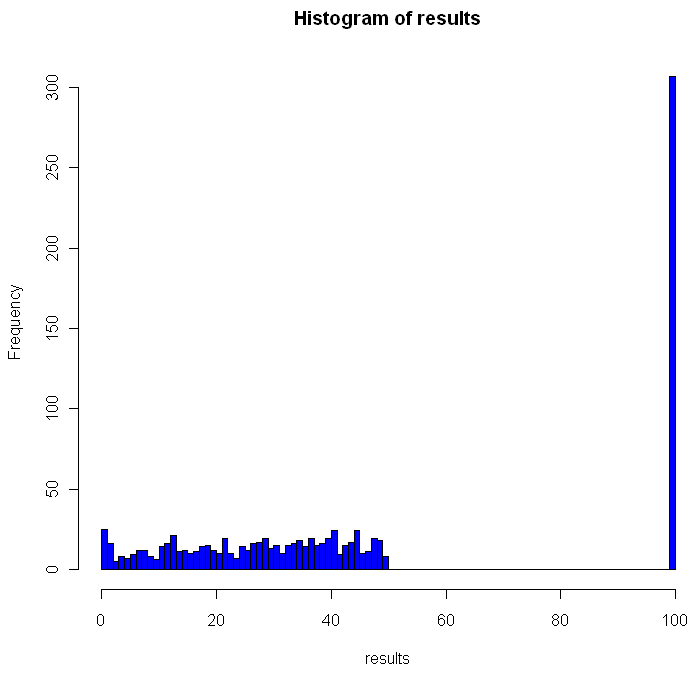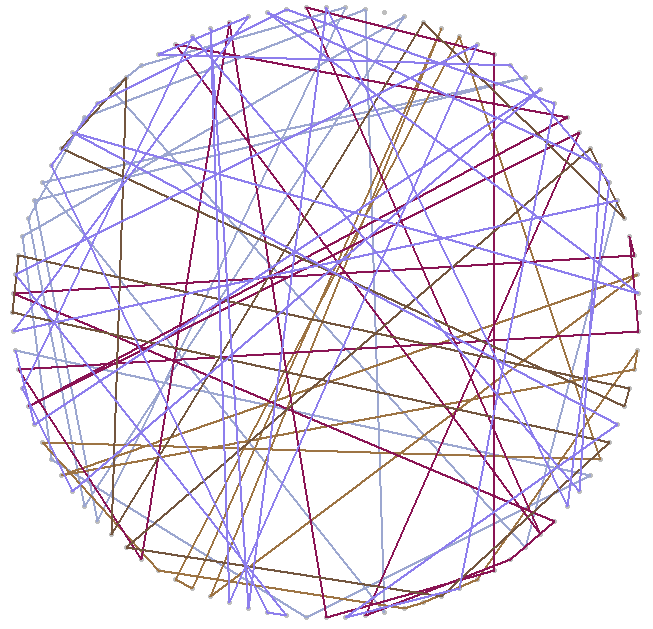[This article was first published on Probability and statistics blog » r, and kindly contributed to R-bloggers]. (You can report issue about the content on this page here)
Want to share your content on R-bloggers? click here if you have a blog, or here if you don't.In math and economics, there is a long, proud history of placing imaginary prisoners into nasty, complicated scenarios. We have, of course, the classic Prisoner’s Dilemma, as well as 100 prisoners and a light bulb. Add to that list the focus of this post, 100 prisoners and 100 boxes.

In this game, the warden places 100 numbers in 100 boxes, at random with equal probability that any number will be in any box. Each convict is assigned a number. One by one they enter the room with the boxes, and try to find their corresponding number. They can open up to 50 different boxes. Once they either find their number or fail, they move on to a different room and all of the boxes are returned to exactly how they were before the prisoner entered the room.

The prisoners can communicate with each other before the game begins, but as soon as it starts they have no way to signal to each other. The warden is requiring that all 100 prisoners find their numbers, otherwise she will force them to listen to hundreds of hours of non-stop, loud rock musician interviews. Can they avoid this fate?

The first thing you might notice is that if every prisoner opens 50 boxes at random, they will have a$0.5$ probability of finding their number. The chances that all of them will find their number is$(frac{1}2)^{100}$, which is approximately as rare as finding a friendly alien with small eyes. Can they do better?

Of course they can. Otherwise I wouldn’t be asking the question, right? Before I explain how, and go into a Monte Carlo simulation in R, you might want to think about how they can do it. No Googling!

All set? Did you find a better way? The trick should be clear from the code below, but if not skip on to the explanation.

```# How many times should we run this experiment?
iters = 1000
results = rep(0,iters)

for(i in 1:iters) {
# A random permutation:
boxes = sample(1:100,100)

# Labels for our prisoners
prisoners = 1:100

# Track how many "winners" we have
foundIt = 0

# Main loop over the prisoners
for(prisoner in prisoners) {

# Track the prisoners path
path = c(prisoner)

tries = 1

# Look first in the box that matches your own number
inBox = boxes[prisoner]

while(tries < 50) { 			 			path = c(path, inBox) 			 			if(inBox == prisoner) { 				#cat("Prisoner", prisoner, "found her number on try", tries, "n") 				foundIt = foundIt + 1 				break; 			} else { 				# Follow that number to the next box 				inBox = boxes[inBox] 			} 			tries = tries+1 		} 		 		# cat("Prisoner", prisoner, "took path", paste(path, collapse=" -> "), "n")
}

# How many prisoners found their numbers?
cat("A total of", foundIt, "prisoners found their numbers.n")
flush.console()
results[i] = foundIt
}

hist(results, breaks=100, col="blue")```

Here is what one of my plots looked like after running the code:Out of the 1000 times I ran the experiment, on 307 occasions every single prisoner found his number. The theoretical success rate is about 31%. So, if it’s not clear from the code, what was the strategy employed by the prisoners and how does it work?

One way to look at the distribution of numbers in boxes is to see it as a permutation of the numbers from 1 to 100. Each permutation can be partitioned into what are called cycles. A cycle works like this: pick any number in your permutation. Let’s say it’s 23. Then you look at the number the 23rd place (ie the number in the 23rd box, counting from the left). If that number is 16, you look at the number in the 16th place. If that number is 87, go open box number 87 and follow that number. Eventually, the box you open up will have the number that brings you back to where you started, completing the cycle. Different permutations have different cycles.

The key for the prisoner is that by starting with the box that is the same place from the left as his number, and by following the numbers in the boxes, the prisoner guarantees that if he is in a cycle of length less than 50, he will eventually open the box with his number in it, which would complete the cycle he began. One way to envision cycles of different lengths is to think about the extreme cases. If a particular permutation shifted every single number over one to the left (and wrapped number 1 onto the end), you would have a single cycle of length 100. Box 1 would contain number 2, box 2 number 3 and so on. On the other hand, if a permutation flipped every pair of consecutive numbers, you would have 50 cycles, each of length 2: box 1 would have number 2, box 2 would have number 1. Of course if your permutation doesn’t change anything you have 100 cycles of length 1.

As you can see from the histogram, when using this strategy you can never have between 50 and 100 winning prisoners. Anytime you have a single cycle of length greater than 50, for example 55, then all 55 prisoners who start on that cycle will fail to find their number. If no cycles are longer than 50, everyone wins. Just how rare are different cycles of different lengths? For the math behind that check out this excellent explanation by Peter Taylor of Queen’s University.

Before moving on I wanted to visualize these cycles. Try running the code below:

```# Unit circle
plot(0,0,xlim=c(-1,1),ylim=c(-1,1),col="white",ann=FALSE, xaxt="n", yaxt="n")
for(i in 1:100) {
points(cos(2*i/100*pi), sin(2*i/100*pi),pch=20,col="gray")
}

mySample = sample(1:100,100)
for(i in 1:100) {
found = FALSE
nextItem = i

# Pick a random color for this cycle
color = sample(c(0:9,"A","B","C","D","E","F"),12,replace=T)
lineColor = paste("#", paste(color[1:6],collapse=""),sep="")

while(!found) {
# Draw the cycle

segments(cos(nextItem/50*pi), sin(nextItem/50*pi), cos(mySample[nextItem]/50*pi), sin(mySample[nextItem]/50*pi),col=lineColor,lwd=2)
Sys.sleep(.4)
if(mySample[nextItem] == i) {
found = TRUE
} else {
nextItem = mySample[nextItem]
}
}
}```

You can adjust the “Sys.sleep()” parameter to make the animation faster. I recommend running the code to see how the cycles “develop” over time, but here’s a snapshot of what I got: